MPM 1 D Course Review Grade 9 Review

• Slides: 18MPM 1 D – Course Review Grade 9 Review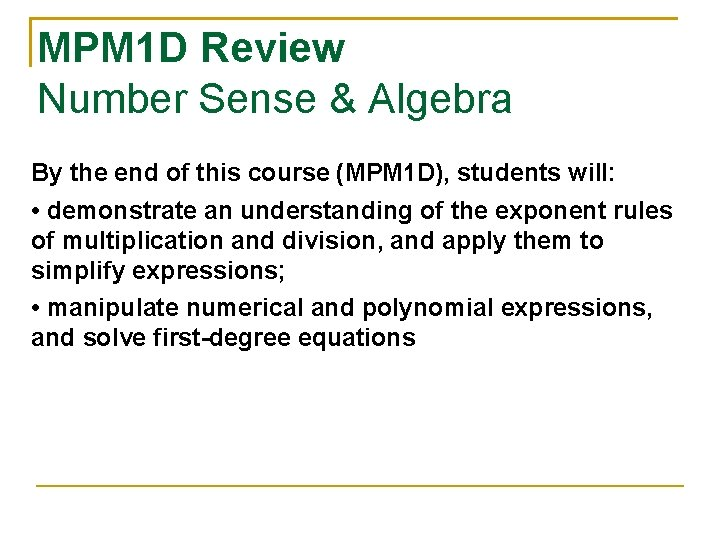MPM 1 D Review Number Sense & Algebra By the end of this course (MPM 1 D), students will: • demonstrate an understanding of the exponent rules of multiplication and division, and apply them to simplify expressions; • manipulate numerical and polynomial expressions, and solve first-degree equations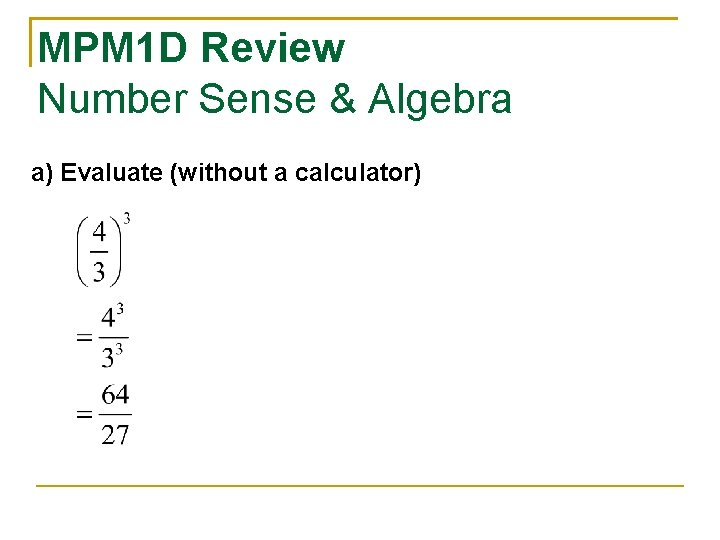MPM 1 D Review Number Sense & Algebra a) Evaluate (without a calculator)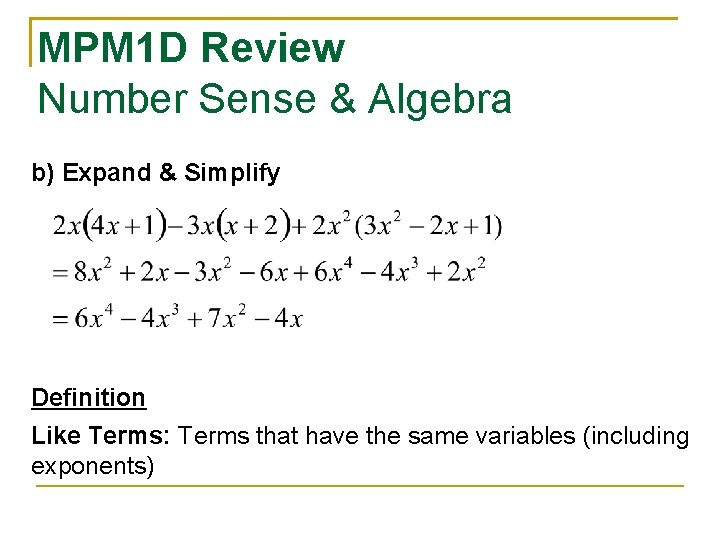MPM 1 D Review Number Sense & Algebra b) Expand & Simplify Definition Like Terms: Terms that have the same variables (including exponents)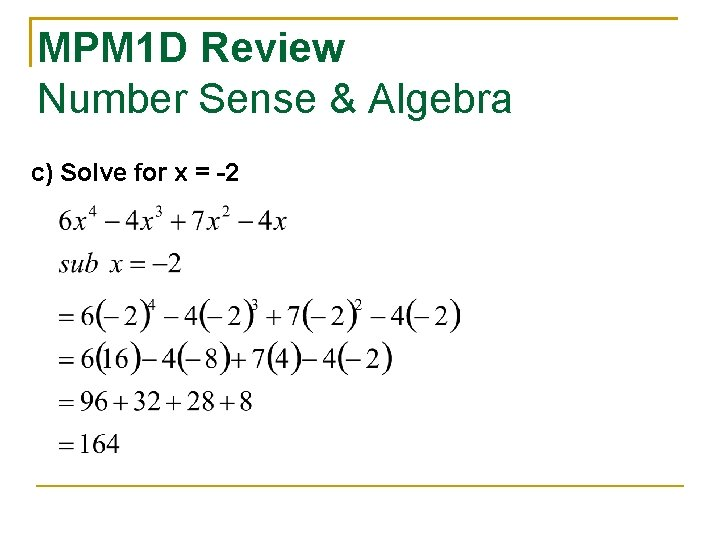MPM 1 D Review Number Sense & Algebra c) Solve for x = -2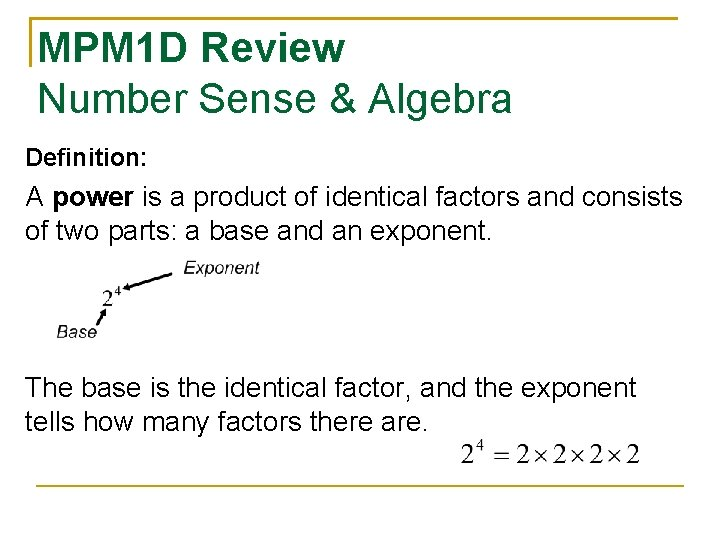MPM 1 D Review Number Sense & Algebra Definition: A power is a product of identical factors and consists of two parts: a base and an exponent. The base is the identical factor, and the exponent tells how many factors there are.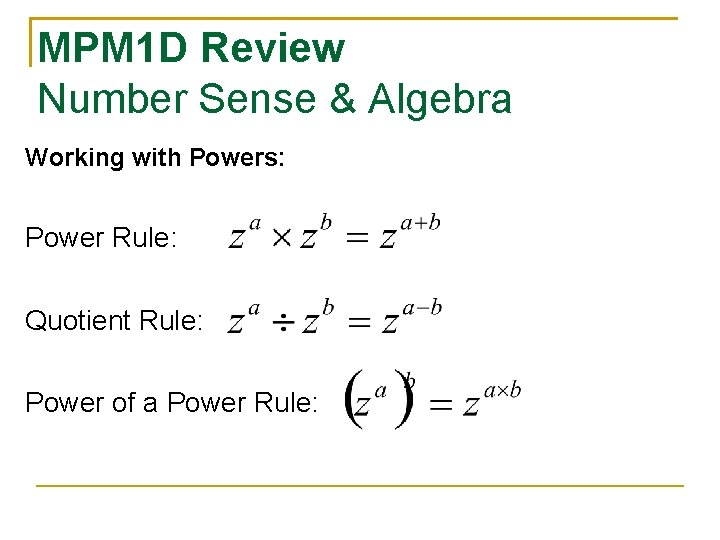MPM 1 D Review Number Sense & Algebra Working with Powers: Power Rule: Quotient Rule: Power of a Power Rule: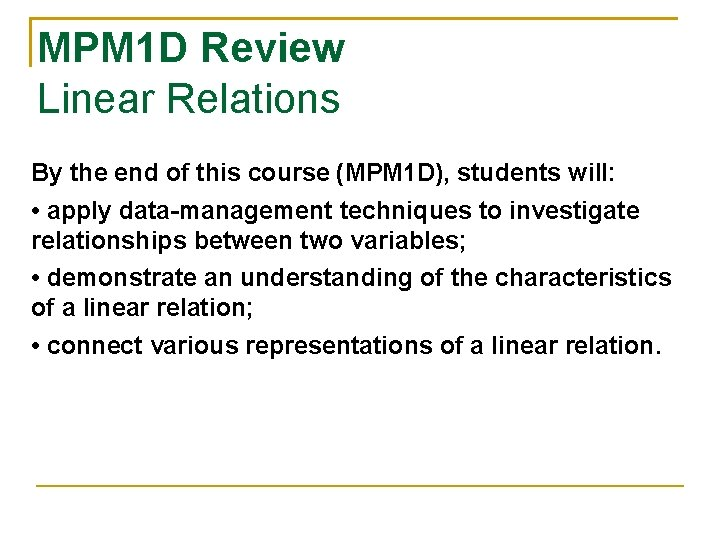MPM 1 D Review Linear Relations By the end of this course (MPM 1 D), students will: • apply data-management techniques to investigate relationships between two variables; • demonstrate an understanding of the characteristics of a linear relation; • connect various representations of a linear relation.MPM 1 D Review Linear Relations Linear Representations 1) Equation 2) Table of Values 3) Graph 4) Words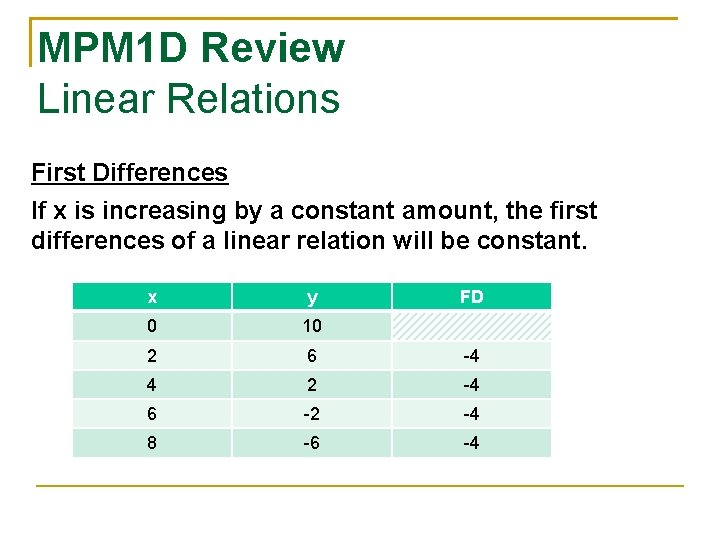MPM 1 D Review Linear Relations First Differences If x is increasing by a constant amount, the first differences of a linear relation will be constant. x y FD 0 10 2 6 -4 4 2 -4 6 -2 -4 8 -6 -4MPM 1 D Review Analytic Geometry By the end of this course (MPM 1 D), students will: • determine the relationship between the form of an equation and the shape of its graph with respect to linearity and non-linearity; • determine, through investigation, the properties of the slope and y-intercept of a linear relation; • solve problems involving linear relations.MPM 1 D Review Analytic Geometry Equation of a Line (slope/y-int form) x: input values y: output values m: slope / rate of change b: y-int (value of y when x = 0)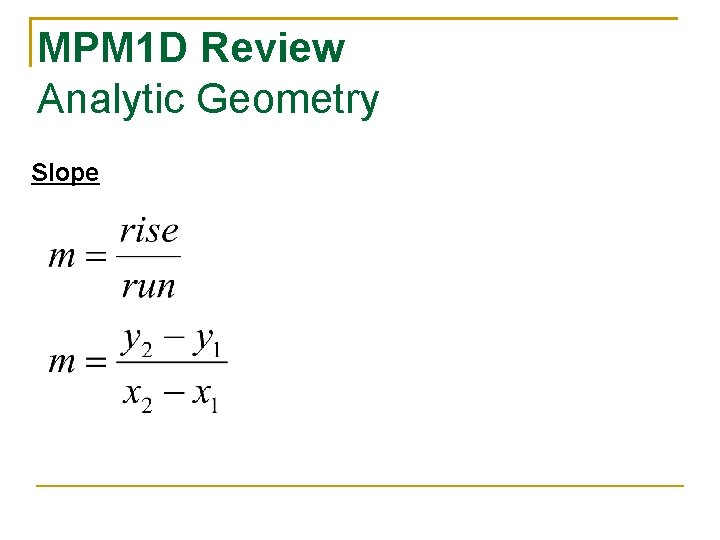MPM 1 D Review Analytic Geometry Slope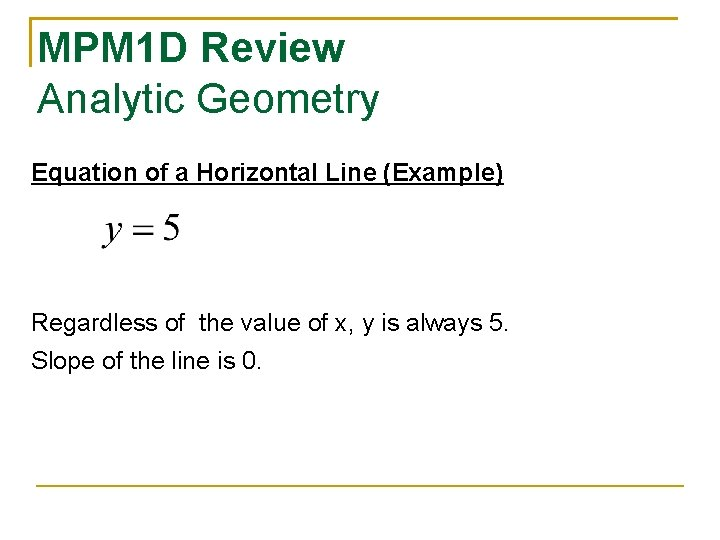MPM 1 D Review Analytic Geometry Equation of a Horizontal Line (Example) Regardless of the value of x, y is always 5. Slope of the line is 0.MPM 1 D Review Analytic Geometry Equation of a Vertical Line (Example) Regardless of the value of y, x is always 4. Slope of the line is undefined.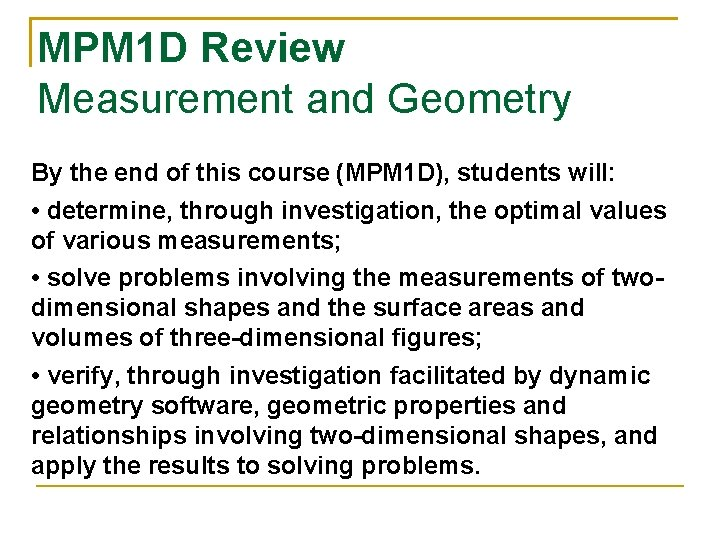MPM 1 D Review Measurement and Geometry By the end of this course (MPM 1 D), students will: • determine, through investigation, the optimal values of various measurements; • solve problems involving the measurements of twodimensional shapes and the surface areas and volumes of three-dimensional figures; • verify, through investigation facilitated by dynamic geometry software, geometric properties and relationships involving two-dimensional shapes, and apply the results to solving problems.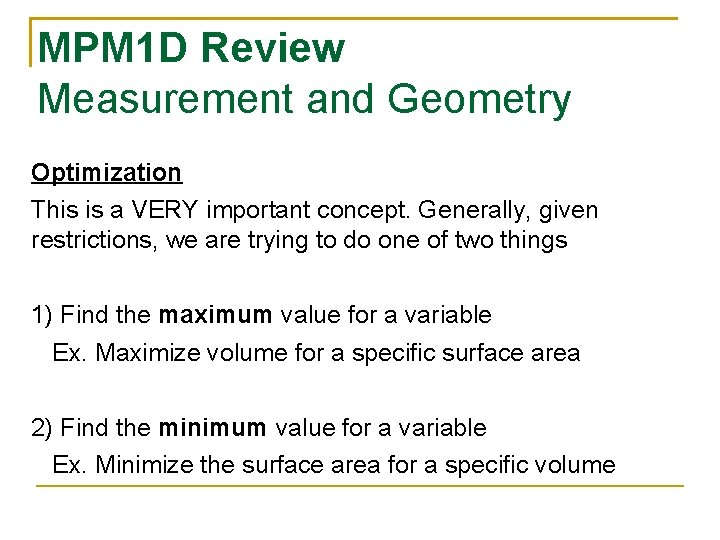MPM 1 D Review Measurement and Geometry Optimization This is a VERY important concept. Generally, given restrictions, we are trying to do one of two things 1) Find the maximum value for a variable Ex. Maximize volume for a specific surface area 2) Find the minimum value for a variable Ex. Minimize the surface area for a specific volume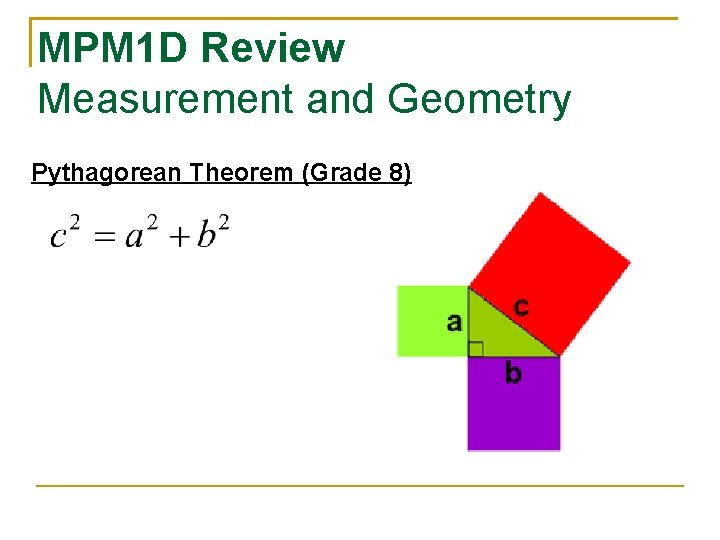MPM 1 D Review Measurement and Geometry Pythagorean Theorem (Grade 8)